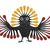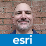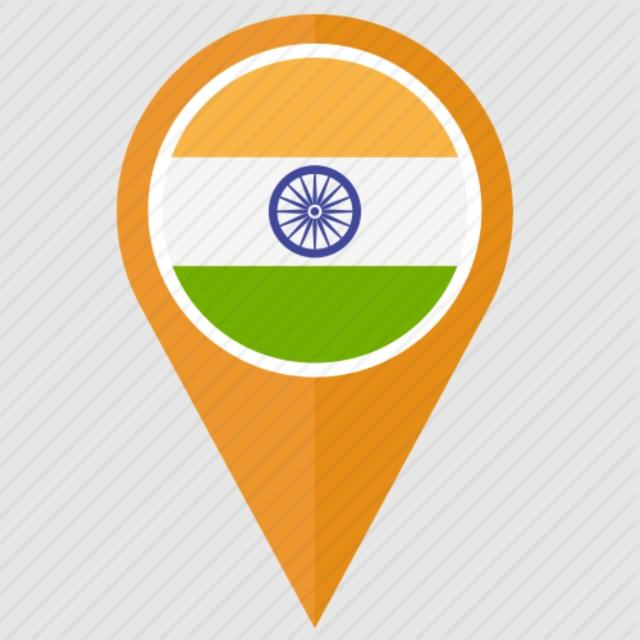# Split a polygon into percentage of total surface

709
3
07-21-2021 12:45 PMby
Occasional Contributor

Hi Esri community,

I want to split a polygon into the percentage of its total surface. For example, the polygon is 5000km2 and I want to extract 30%, 20% and 50% of the total surface. How can I make a split based on a percentage of the surface?

Anyone has a solution? Thanks in advance

Isabelle

3 RepliesbyEsri Esteemed Contributor

There are an infinite number of ways to slice a polygon. Actually doing so with a specific ratio to previous area is an "NP Hard" or possibly "NP Complete" problem ("NP" comes from "nondeterministic polynomial-time", in the domain of computational complexity theory , basically, that there's no way to predict how long it will take, even if you know you have the problem partly solved).

All the possible solutions are iterative in nature, and very expensive, computationally. If the polygons are multipart (islands) or contain subparts (holes), the complexity of the problem increases (since the area of shifting slices could go down for a while, then back up again, making binary search algorithms inoperative).

If you have a particular dimension for which you want to optimize, you can start that way, but the best you may be able to accomplish is to arrive, after an inordinate amount of processing, with an answer "close enough" to quit trying any more.

This is a problem where human vision and intuition could simplify the solution, at the cost of needing a user to review every geometry, and start the process or wave off continued effort from a given end point.

- VbyMVP Esteemed Contributor

Here is a workflow I can think of (assuming your example).

Use Subdivide Polygon to divide it into 10 equal parts(Subdivision method= No. of Equal Parts) each having 10% of the area.

Using Editing tool, Select and Merge 3 polygons, 2 polygons and 5 polygons respectively. This will result in 3 polygon parts of 30%, 20% and 50% respectively.

Think LocationbyMVP Esteemed Contributor

It's a cool idea, but like @VinceAngelo suggests, which one do you want? Here are three simple options of splitting a polygon approximately 50/50.

Even with @JayantaPoddar 's suggestion, you are limited to what the software will divide up for you...That should just about do it....